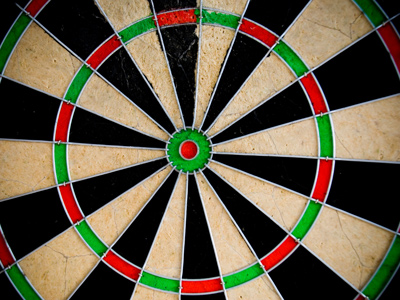On a dartboard scoring a double 18 and a treble 18 results in a score of 90.

# Complete the Sum 4

This Complete the Sum quiz will test you on subtraction.

What you have been doing in this series of 11-plus verbal reasoning quizzes is basic algebra, where a symbol stands in for a number. We hope that when you begin algebra in secondary school you will be ahead of the game.

If you haven't already played our first three quizzes in this series, please do go back and play them first. Only when you have got 40 out of 40 will you be a whizz at maths!

Enjoy the final quiz in the Complete the Sum series. As always, take your time and look at the questions and answers carefully before choosing.

Example:

26 + 7 = 18 + ?

13
14
15
16

This is because 26 + 7 = 33. 18 + ? = 33. This makes 15 the only possible answer.
1.
Find the number that will complete the sum below correctly.

27 - 13 = 42 ÷ ?
5
6
4
3
This is because 27 - 13 = 14. 42 ÷ 3 is also equal to 14. The first three square numbers are 1, 4 and 9. 14 is the sum of these square numbers
2.
Find the number that will complete the sum below correctly.

63 ÷ 9 = 32 - ?
24
25
26
27
This is because 32 - 25 = 7 and 63 ÷ 9 = 7
3.
Find the number that will complete the sum below correctly.

4 x 16 = ? - 55
78
117
119
55
This is because 119 - 55 = 64, which is the same as 4 x 16. That was one to get your brain working a little harder! Did it work, or are you an expert at these questions now?
4.
Find the number that will complete the sum below correctly.

79 + ? = 9 x 9
2
4
7
9
This is because 9 x 9 = 81. To get to this product from 79 we have to add 2
5.
Find the number that will complete the sum below correctly.

56 ÷ 8 = ? - 8
13
19
17
15
This is because 56 ÷ 8 = 7. This is the same as 15 - 8. A cubed number is a number multiplied by itself and then by itself again (for example, 3 x 3 x 3 = 27, making 27 the cube of 3 and 3 the cube root of 27). Can you work out the cube root of 8? Read below for the answer
6.
Find the number that will complete the sum below correctly.

39 ÷ 3 = ? - 18
13
33
31
18
This is because 39 ÷ 3 = 13, which is equal to 31 - 18
7.
Find the number that will complete the sum below correctly.

? - 45 = 8 x 4
77
75
73
71
This is because 77 - 45 = 32 and 8 x 4 = 32. Remember the challenge we set you in question 5? Did you work out that the cube root of 8 is 2?
8.
Find the number that will complete the sum below correctly.

72 - 14 = ? x 2
28
26
27
29
This is because 29 x 2 = 58. Also, 72 - 14 = 58
9.
Find the number that will complete the sum below correctly.

? ÷ 13 = 90 ÷ 18
67
66
65
69
This is because 90 ÷ 18 = 5 and 65 ÷ 13 = 5. Only 65 works. On a dartboard scoring a double 18 and a treble 18 results in a score of 90. What would a treble and a double 19 score? Read below for the answer
10.
Find the number that will complete the sum below correctly.

27 x 3 = 9 x ?
11
7
8
9
This is because 27 x 3 = 81 and 9 x 9 also equals 81. The answer to the little quiz in question 9 is 95 (19 x 3 = 57 and 19 x 2 = 38. 57 + 38 = 95). We hope you enjoyed this series of quizzes, and look forward to you trying the next series of Verbal Reasoning quizzes!
Author:  Stephen O'Hara